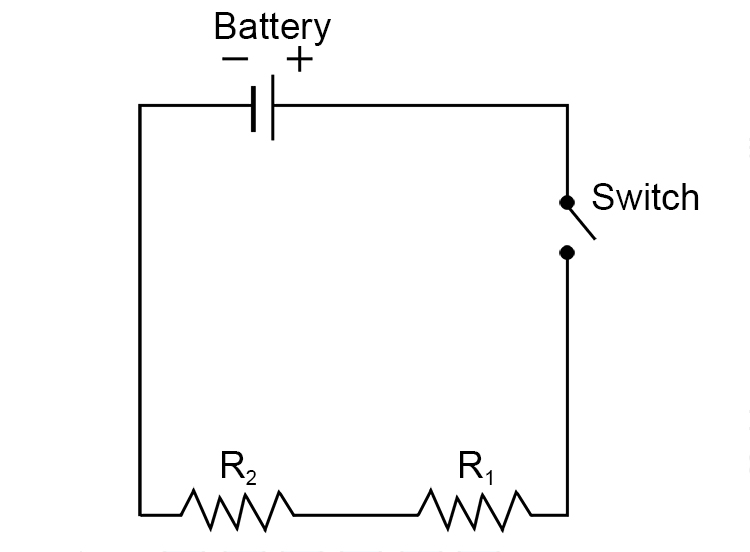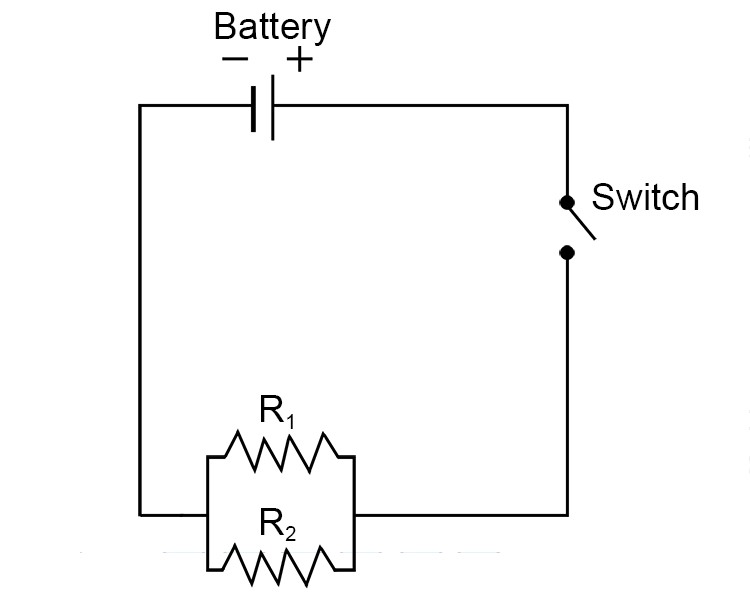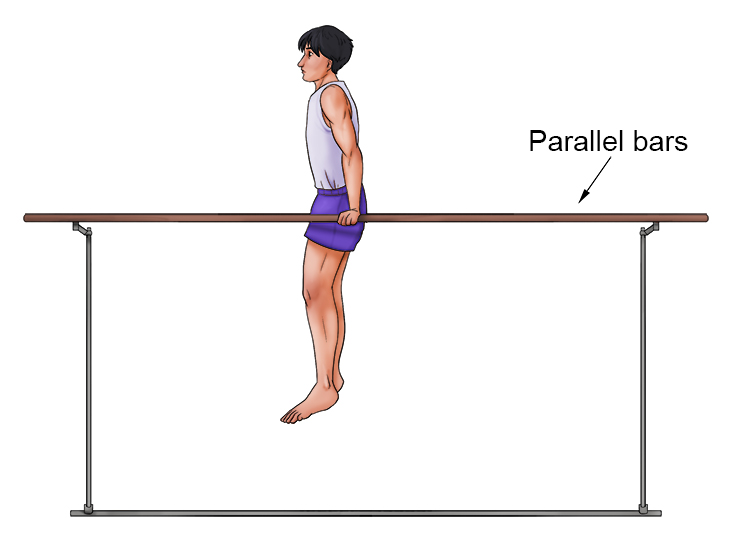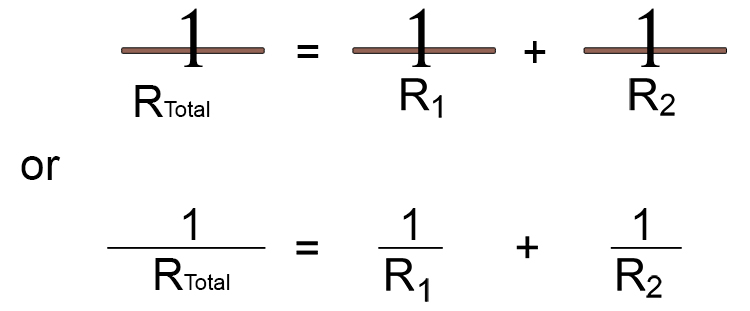# Series and Parallel Circuits Resistance Total Mnemonic

A series circuit of resistances, like a light bulb, are connected in line with each other.The total resistance is easy to remember.

R_{Total}=R_1+R_2

Total Resistance = Resistance 1 + Resistance 2

But what about when they are parallel?Use the mnemonic or aid below to remind you that you are dealing with something parallel, similar to the parallel bars in gymnastics.

Parallel = Parallel Bars

Which looks like this from the side:But think of the man being a number 1 instead.Use this image to put parallel bars in the formula for resistance. Instead of

R_{Total}=R_1+R_2

Now put them as parallel resistance and add parallel bars.Summary

1/ Resistance in series

\text{Total Resistance in series}\text{ }=R_1+R_2

2/ Resistance in parallel

\frac{1}{\text{Total Resistance in Parallel}}=\frac{1}{R_1}+\frac{1}{R_2}